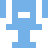### Real Vim ninjas count every keystroke - do you?

###### Pick a challenge, fire up Vim, and show us what you got.

```Your VimGolf key: please sign in

\$ gem install vimgolf
\$ vimgolf setup
\$ vimgolf put 5f1310bd3cc9f3000bef87ed
```

### Levenshtein distance

Compute distance for each pair. Notice that this recursive implementation is very inefficient. Wagner-Fischer algorithm is iterative and much faster. #vimscript

##### Start file
```" Levenshtein distance between two strings
function! LD(s1, s2)
let l1 = strlen(a:s1)
let l2 = strlen(a:s2)
if l1 <= 0
return l2
elseif l2 <= 0
return l1
elseif a:s1 ==# a:s2
return LD(a:s1[1:], a:s2[1:])
else
return 1 + min([
\ LD(a:s1, a:s2[1:]), LD(a:s1[1:], a:s2), LD(a:s1[1:], a:s2[1:])
\ ])
endif
endfunction
" Humm... Is this useful?
"nmap M I<C-R>=LD("<C-R>a","<C-R>b")<CR><Esc>

"VIM VimGolf
"keystroke matt
"vimscript way
"reformat refactor
"challenge chatter
"colons semicolons
"golfer golfers
"order sorting
"modules models
"shift+ret ctrl+ret
"ninja manic
"destination distance
"pattern lines
"linewrapping linewrapping
"swap switch
"vice versa
"split multiples
"block blackened
"-a-b-c c-a-b
"hello_world hello world!
```
```6
8
9
5
5
4
1
5
2
5
4
7
6
0
4
4
7
5
3
2
```

#### View Diff

```1,39c1,20
< " Levenshtein distance between two strings
< function! LD(s1, s2)
<   let l1 = strlen(a:s1)
<   let l2 = strlen(a:s2)
<   if l1 <= 0
<     return l2
<   elseif l2 <= 0
<     return l1
<   elseif a:s1 ==# a:s2
<     return LD(a:s1[1:], a:s2[1:])
<   else
<     return 1 + min([
<     \ LD(a:s1, a:s2[1:]), LD(a:s1[1:], a:s2), LD(a:s1[1:], a:s2[1:])
<     \ ])
<   endif
< endfunction
< " Humm... Is this useful?
< "nmap M I<C-R>=LD("<C-R>a","<C-R>b")<CR><Esc>
<
< "VIM VimGolf
< "keystroke matt
< "vimscript way
< "reformat refactor
< "challenge chatter
< "colons semicolons
< "golfer golfers
< "order sorting
< "modules models
< "shift+ret ctrl+ret
< "ninja manic
< "destination distance
< "pattern lines
< "linewrapping linewrapping
< "swap switch
< "vice versa
< "split multiples
< "block blackened
< "-a-b-c c-a-b
< "hello_world hello world!
---
> 6
> 8
> 9
> 5
> 5
> 4
> 1
> 5
> 2
> 5
> 4
> 7
> 6
> 0
> 4
> 4
> 7
> 5
> 3
> 2
```